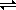# 15.11: The Solubility-Product Constant

Skills to Develop

• Understand the basic of solution-solid equilibria

We will now return to an important mathematical relationship we first learned about in our unit on Equilibrium, the equilibrium constant expression. Recall that for any general reaction:

$aA + bB \rightleftharpoons cD + dD$

an equilibrium constant expression can be defined as:

$K_{eq} = \dfrac{[C]^c[D]^d}{[A]^a[B]^b}$

Since saturated solutions are equilibrium systems, we can apply this mathematical relationship to solutions. We will refer to our equilibrium constant as $$K_{sp}$$, where "sp" stands for "solubility product"

For our silver sulfate saturated solution,

$Ag_2SO_{4 (s)} \rightleftharpoons 2Ag^+_{(aq)} + SO^{2-}_{4(aq)}$

we can write a solubility product constant expression as

$K_{sp} = \dfrac{ [Ag^+_{(aq)}]^2[SO_{4(aq)}^{2-}] }{ [Ag_2SO_{4(s)}] }$

However, remember from our earlier introduction to the equilibrium constant expression that the concentrations of solids and liquids are NOT included in the expression because while their amounts will change during a reaction, their concentrations will remain constant. Therefore, we will write our solubility product constant expression for our saturated silver sulfate solution as:

$K_{sp}= [Ag^+]^2[SO_4^{2-}]$

Please note that it is VERY IMPORTANT to include the ion charges when writing this equation.

Example $$\PageIndex{1}$$

Write the expression for the solubility product constant, Ksp, for Ca3(PO4)2.

Solution

Step 1: Begin by writing the balanced equation for the reaction. Remember that polyatomic ions remain together as a unit and do not break apart into separate elements.

$Ca_3(PO_4)_{2 (s)} \rightleftharpoons 3 Ca^{2+}_{(aq)} + 2 PO^{3-}_{4(aq)}$

Step 2: Write the expression for $$K_{sp}$$:

$K_{sp}= [Ca^{2+}]^3[PO_4^{3-}]^2$

Solubility Product Tables that give Ksp values for various ionic compounds are available. Because temperature affects solubility, values are given for specific temperatures (usually 25°C). Recall what we learned about Keq

• If Keq is very large, the concentration of the products must be much greater than the concentration of the reactants. The reaction essentially "goes to completion", which all - or most of - the reactants being used up to form the products.
• If Keq is very small, the concentration of the reactants is much greater than the concentration of the products. The reaction does not occur to any great extent - most of the reactants remain unchanged, and there are few products produced.
• When Keq is not very large or very small, then roughly equal amounts of reactants and products are present at equilibrium.

$$K_{sp}$$, which again is just a special case of $$K_{eq}$$, provides us with the same useful information:

A low value of Ksp indicates a substance that has a low solubility (it is generally insoluble); for ionic compounds this means that there will be few ions in solution.

• Iron(II) sulfide, FeS, is an example of a low Ksp : Ksp = 4 ×10-19. In a saturated solution of FeS there would be few Fe2+ or S2- ions.

A large value of Ksp indicates a soluble substance; for ionic compounds it tells us that there will be many ions in solution.

• An example of a relatively large Ksp would be for lead(II) chloride, PbCl2 which has a Ksp of 1.8 ×10-4. A saturated solution of PbCl2 would have a relatively high concentration of Pb 2+ and Cl - ions.

There are several types of problems we can solve:

Example $$\PageIndex{1}$$: Calculating Ksp of a Saturated Solution

The concentration of a saturated solution of BaSO4 is 3.90 × 10-5M. Calculate Ksp for barium sulfate at 25°C

Solution

Always begin problems involving Ksp by writing a balanced equation:

BaSO4 (s)Ba2+(aq) + SO42- (aq)

Next, write the Ksp expression:

Ksp= [Ba2+][SO42-]

The question provides us with the concentration of the solution, BaSO4 . We need to find the concentration of the individual ions for our equation.

Recall from Section 2.5 - since 1 mole of BaSO4 produces 1 mole of Ba2+ and also 1 mole of SO42-, then . . .

• [BaSO4] = 3.90 × 10-5M
• [Ba2+] = 3.90 × 10-5M
• [SO42-] = 3.90 × 10-5M

Substitute values into the Ksp expression and solve for the unknown:

$K_{sp} = [Ba^{2+}][SO_4^{2-}]$

$= (3.90 \times 10^{-5})(3.90 \times 10^{-5})$

$= 1.52 \times 10^{-9}$

Example $$\PageIndex{3}$$: Calculating ion concentrations when Ksp is known

The $$K_{sp}$$ for MgCO3 at 25°C is 2.0 × 10-8. What are the ion concentrations in a saturated solution at this temperature?

Solution

As always, begin with a balanced equation:

MgCO3(s)Mg2+(aq) + CO32-(aq)

Write the Ksp expression:

$K_{sp}= [Mg^{2+}][CO_3^{2-}]$

For this example, we are given the value for Ksp and need to find the ion concentrations.

We will let our unknown ion concentrations equal x.

The balanced equation tells us that both Mg2+ and CO32- will have the same concentration!

Substitute values into the equation and solve for the unknown

Ksp = [Mg2+][CO32-]

x2 = 2.0 × 10-8

x = √(2.0 × 10-8)

find the square root of 2.0 × 10-8

= 1.4× 10-4M

x = [Mg2+]

= 1.4× 10-4M